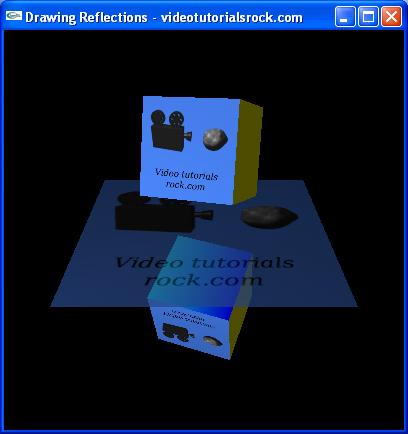# Lesson 14: Drawing Reflections

## Reflections

The basic idea behind making reflections is to draw the object or objects that you want to be reflected across the surface, then to draw a second, reflected copy of the objects. Then, you can use alpha blending to blend the reflective surface onto the screen. So let's do that. Let's take the cube from this lesson, draw two copies of it, and blend a floor to the screen.Oops. Our cube has "leaked" off of the reflective surface. We're actually going to need to understand a new concept: the stencil buffer.

The stencil buffer gives us a certain amount of memory for each pixel. In this program, we're only going to need one bit per pixel. In order to make the reflected part of the cube, we're first going to set every pixel covered by the floor to be 1 in the stencil buffer. Then, we'll draw the reflected version of the cube only where the stencil buffer is 1. After doing this, we get the following outcome:Aah. Much better.

## The Code

It's that time again: time to run through the code.

```const float BOX_SIZE = 7.0f; //The length of each side of the cube
const float BOX_HEIGHT = BOX_SIZE; //The height of the box off of the ground
const float FLOOR_SIZE = 20.0f; //The length of each side of the floor```

We have some constants, explained by their comments.

```//Draws the cube
void drawCube(int textureId, float angle) {
//...
}

//Draws the floor
void drawFloor(int textureId) {
//...
}```

We have drawCube and drawFloor functions. I'm sure you can guess what they do.

```void drawScene() {
glClear(GL_COLOR_BUFFER_BIT | GL_DEPTH_BUFFER_BIT | GL_STENCIL_BUFFER_BIT);```

We have a little something new at the top of drawScene. In addition to GL_COLOR_BUFFER_BIT and GL_DEPTH_BUFFER_BIT, we're adding GL_STENCIL_BUFFER_BIT to the call to glClear. This makes it so that we clear the stencil buffer, so every pixel in the stencil buffer is equal to 0.

```    //...
glPushMatrix();
glTranslatef(0, BOX_HEIGHT, 0);
drawCube(_textureId, _angle);
glPopMatrix();```

With this code, we draw the normal copy of the cube.

```    glEnable(GL_STENCIL_TEST); //Enable using the stencil buffer
glColorMask(0, 0, 0, 0); //Disable drawing colors to the screen
glDisable(GL_DEPTH_TEST); //Disable depth testing
glStencilFunc(GL_ALWAYS, 1, 1); //Make the stencil test always pass
//Make pixels in the stencil buffer be set to 1 when the stencil test passes
glStencilOp(GL_KEEP, GL_KEEP, GL_REPLACE);
//Set all of the pixels covered by the floor to be 1 in the stencil buffer
drawFloor(_textureId);```

In this section of code, we're going to set every pixel covered by the floor to be 1 in the stencil buffer. First, we enable GL_STENCIL_TEST to indicate that we want to start using the stencil buffer. Then, we call glColorMask(0, 0, 0, 0) to disable drawing to the screen. Right now, we only want to draw to the stencil buffer, not to the screen. Then, we disable GL_DEPTH_TEST, since we don't need depth testing, in order to speed things up a little.

There are actually lots of interesting things you can do with stencil buffers, but I'll only explain what we need to know for reflections. The call to glStencilFunc(GL_ALWAYS, 1, 1) makes it so that the stencil test always passes. I'll explain a little about the stencil test later. Then, we call glStencilOp(GL_KEEP, GL_KEEP, GL_REPLACE), which makes it so that every pixel where we draw will be set to 1 in the stencil buffer. As a result, the subsequent call to drawFloor has the effect of setting every pixel that the floor occupies to be 1 in the stencil buffer. And now, we're all set. The stencil buffer is 1 where the floor is and 0 everywhere else.

```    glColorMask(1, 1, 1, 1); //Enable drawing colors to the screen
glEnable(GL_DEPTH_TEST); //Enable depth testing
//Make the stencil test pass only when the pixel is 1 in the stencil buffer
glStencilFunc(GL_EQUAL, 1, 1);
glStencilOp(GL_KEEP, GL_KEEP, GL_KEEP); //Make the stencil buffer not change

//Draw the cube, reflected vertically, at all pixels where the stencil
//buffer is 1
glPushMatrix();
glScalef(1, -1, 1);
glTranslatef(0, BOX_HEIGHT, 0);
drawCube(_textureId, _angle);
glPopMatrix();```

Now, we need to draw the reflected copy of the cube. We call glColorMask(1, 1, 1, 1) to re-enable drawing to the screen, since we actually want the reflection to show up on the screen. We also re-enable depth testing. We call glStencilFunc(GL_EQUAL, 1, 1), which makes it so that the stencil test only passes at pixels where the stencil buffer is 1. OpenGL will only draw the reflection where this test passes. Then, we call glStencilOp(GL_KEEP, GL_KEEP, GL_KEEP), which makes it so that we won't alter the stencil buffer while drawing the reflection. Then, we actually draw the reflection, using a call to drawCube.

```    glDisable(GL_STENCIL_TEST); //Disable using the stencil buffer

//Blend the floor onto the screen
glEnable(GL_BLEND);
glColor4f(1, 1, 1, 0.7f);
drawFloor(_textureId);
glDisable(GL_BLEND);```

We disable stencil testing now that we're done with the stencil buffer. Then, we blend the floor onto the screen, using an opacity of 70%.

And we're done. We've successfully fixed reflection, so that the floor appears to be a reflective surface.

Next is "Lesson 15: Fog".